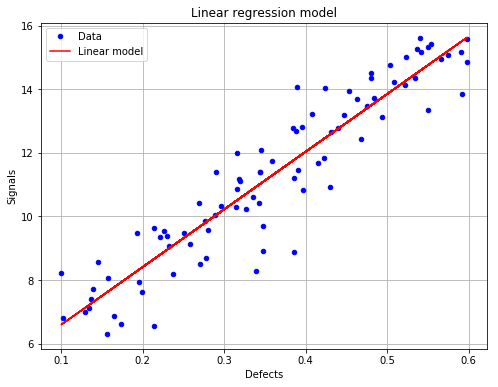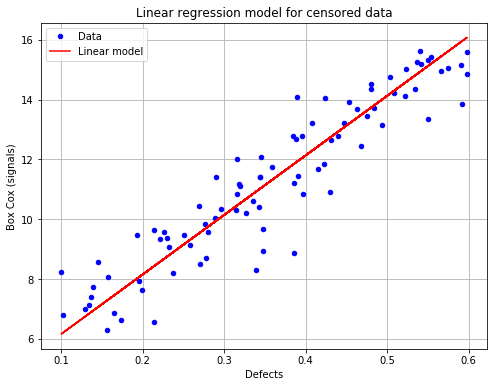# Linear model analysis with censored data¶

:

# import relevant module
import openturns as ot
import otpod
# enable display figure in notebook
try:
%matplotlib inline
except:
pass

/calcul/home/dumas/anaconda/lib/python3.6/site-packages/sklearn/ensemble/weight_boosting.py:29: DeprecationWarning: numpy.core.umath_tests is an internal NumPy module and should not be imported. It will be removed in a future NumPy release.
from numpy.core.umath_tests import inner1d


## Generate data¶

:

N = 100
ot.RandomGenerator.SetSeed(123456)
defectDist = ot.Uniform(0.1, 0.6)
# normal epsilon distribution
epsilon = ot.Normal(0, 1.9)
defects = defectDist.getSample(N)
signalsInvBoxCox = defects * 43. + epsilon.getSample(N) + 2.5
# Inverse Box Cox transformation
invBoxCox = ot.InverseBoxCoxTransform(0.3)
signals = invBoxCox(signalsInvBoxCox)


## Run analysis with Box Cox¶

:

noiseThres = 60.
saturationThres = 1700.
analysis = otpod.UnivariateLinearModelAnalysis(defects, signals, noiseThres,
saturationThres, boxCox=True)


## Get some particular results¶

Result values are given for both analysis performed on filtered data (uncensored case) and on censored data.

:

print(analysis.getIntercept())
print(analysis.getR2())
print(analysis.getKolmogorovPValue())

[Intercept for uncensored case : 4.777, Intercept for censored case : 4.1614]
[R2 for uncensored case : 0.869115, R2 for censored case : 0.860722]
[Kolmogorov p-value for uncensored case : 0.477505, Kolmogorov p-value for censored case : 0.505919]


## Save all results in a csv file¶

:

analysis.saveResults('results.csv')


## Show graphs¶

### The linear regression model with data for the uncensored case (default case)¶

:

# draw the figure for the uncensored case and save it as png file
fig, ax = analysis.drawLinearModel(name='figure/linearModelUncensored.png')
fig.show()

/calcul/home/dumas/anaconda/lib/python3.6/site-packages/matplotlib/figure.py:459: UserWarning: matplotlib is currently using a non-GUI backend, so cannot show the figure
"matplotlib is currently using a non-GUI backend, "### The linear regression model with data for the censored case¶

:

# draw the figure for the censored case and save it as png file
fig, ax = analysis.drawLinearModel(model='censored', name='figure/linearModelCensored.png')
fig.show()

/calcul/home/dumas/anaconda/lib/python3.6/site-packages/matplotlib/figure.py:459: UserWarning: matplotlib is currently using a non-GUI backend, so cannot show the figure
"matplotlib is currently using a non-GUI backend, "[ ]:



[ ]: